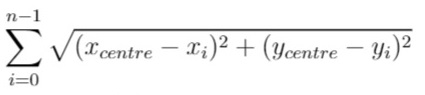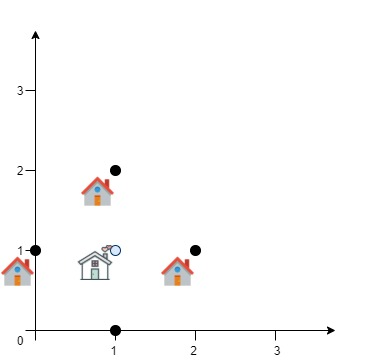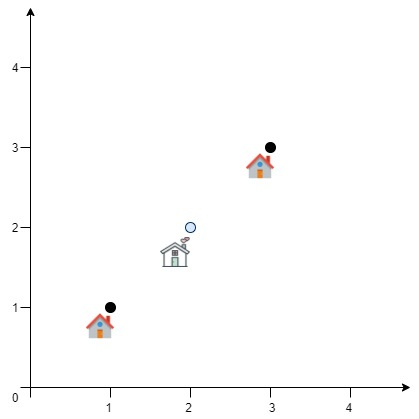# GeetCode Hub

A delivery company wants to build a new service centre in a new city. The company knows the positions of all the customers in this city on a 2D-Map and wants to build the new centre in a position such that the sum of the euclidean distances to all customers is minimum.

Given an array `positions` where `positions[i] = [xi, yi]` is the position of the `ith` customer on the map, return the minimum sum of the euclidean distances to all customers.

In other words, you need to choose the position of the service centre `[xcentre, ycentre]` such that the following formula is minimized:Answers within `10^-5` of the actual value will be accepted.

Example 1:```Input: positions = [[0,1],[1,0],[1,2],[2,1]]
Output: 4.00000
Explanation: As shown, you can see that choosing [xcentre, ycentre] = [1, 1] will make the distance to each customer = 1, the sum of all distances is 4 which is the minimum possible we can achieve.
```

Example 2:```Input: positions = [[1,1],[3,3]]
Output: 2.82843
Explanation: The minimum possible sum of distances = sqrt(2) + sqrt(2) = 2.82843
```

Example 3:

```Input: positions = [[1,1]]
Output: 0.00000
```

Example 4:

```Input: positions = [[1,1],[0,0],[2,0]]
Output: 2.73205
Explanation: At the first glance, you may think that locating the centre at [1, 0] will achieve the minimum sum, but locating it at [1, 0] will make the sum of distances = 3.
Try to locate the centre at [1.0, 0.5773502711] you will see that the sum of distances is 2.73205.
Be careful with the precision!
```

Example 5:

```Input: positions = [[0,1],[3,2],[4,5],[7,6],[8,9],[11,1],[2,12]]
Output: 32.94036
Explanation: You can use [4.3460852395, 4.9813795505] as the position of the centre.
```

Constraints:

• `1 <= positions.length <= 50`
• `positions[i].length == 2`
• `0 <= positions[i], positions[i] <= 100`

class Solution { public double getMinDistSum(int[][] positions) { } }